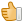# It is possible to implement skinparam features and coloring for different parts of mathematical expressions also?

Let say, for example

skinparam dpi 300

and

color red
f(t)

color black

=(a_0)/2 + sum_(n=1)^ooa_ncos((npit)/L)+sum_(n=1)^oo b_n\ sin((npit)/L)

Anyway thank you for your fast Responseanswered May 30, 2018 by (276,340 points)

For dpi, with last beta http://beta.plantuml.net/plantuml.jar you can have

```@startmath
dpi 300
color red
f(t)=(a_0)/2 + sum_(n=1)^ooa_ncos((npit)/L)+sum_(n=1)^oo b_n\ sin((npit)/L)
@endmath```

About having several colors, the issue is that each color will generate an independant image and it will difficult to align them.

Maybe we could allow:

```@startmath
dpi 300
color red
f(t)
newline
color black
=(a_0)/2 + sum_(n=1)^ooa_ncos((npit)/L)+sum_(n=1)^oo b_n\ sin((npit)/L)
@endmath
```

Do you think it's worth trying ?